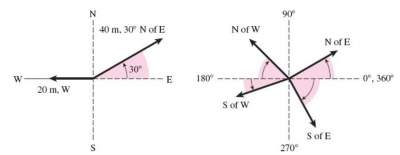# Vector and Scalar Quantities, Vector Addition, and Vector Components

## Vector and Scalar Quantities

### Scalar

A scalar quantity is specified completely by its magnitude, that's a number and a unit.
Examples are speed: 75 km/h, 55 mph; Distance: 50 km, 10 feet; Volume: 500 cm3; 45 feet3.
Scalar quantities that are measured in the same units may be added or subtracted in the usual way.
For example:
23mm + 7mm = 30 mm
54 m2 − 5 m2 = 49 m2

### Vector

A vector quantity is specified completely by a magnitude and a direction.
It consists of a number, a unit, and a direction.
Examples are displacement (35 m, N) and velocity (70 km/h, 30° N of E).
The direction must be a part of any calculations involving vector quantity.
See example below in which the figure shows the direction of a vector by reference to north (N), south (S), east (E), and west (W).Vectors are different from scalars:
1. Vectors must be represented by: a magnitude + direction + units.
Scalars can be represented by only: magnitude + units.
2. Vectors can be added and subtracted using special methods.
Scalars can be added or subtracted simple using algebraic summation.

### Exercise with Solution: Vector or Scalar

 15 m _________________ 55 m/sec, North _________________ 15 km, South _________________ 25 degrees Celsius _________________ 10 exabytes _________________ 100 calories _________________